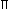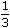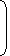# Learn important formulas of Surface Area & Volume in just 2 minutes!#### FORMULAS RELATED TO SURFACE AREA AND VOLUME

• CUBOID : Let length = l, breadth = b and height = h units. Then
1. Volume = (l x b x h) cubic units.
2. Surface area = 2(lb + b h + l h) sq. units.
3. Diagonal =under root( 2 + 2 + 2) units

• CUBE : Let each edge of a cube be of length a. Then,
1. Volume = a3 cubic units.
2. Surface area = 6a2 sq. units.
3. Diagonal = under root  of 3a units.

• CYLINDER : Let radius of base = r and Height (or length) = h. Then,
1. Volume = (r2h) cubic units.
2. Curved surface area = (2rh) sq. units.
3. Total surface area = 2r(h + r) sq. units.

• CONE : Let radius of base = r and Height = h. Then,
1. Slant height, l = h2 + r2 units.
2. Volume =r2hcubic units.
3. Curved surface area = (rl) sq. units.
4. Total surface area = (rl +r2) sq. units.

• SPHERE : Let the radius of the sphere be r. Then,
1. Volume =r3cubic units.
2. Surface area = (4r2) sq. units.

• HEMISPHERE : Let the radius of a hemisphere be r. Then,
1. Volume =r3cubic units.
2. Curved surface area = (2r2) sq. units.
3. Total surface area = (3r2) sq. units.Don't miss out!